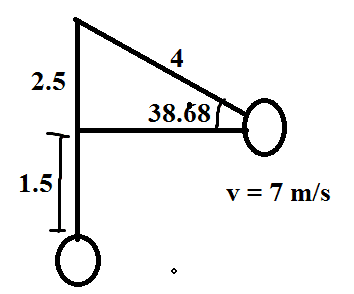# Centripetal Acceleration and Tension: Correct?

## Homework Statement

A stuntman whose mass is 70 kg swings from the end of a 4.0-m-long rope along the arc of a vertical circle. Assuming he starts from rest when the rope is horizontal, find the tensions on the rope that are required to make him follow his circular path at a height of 1.5 m above the bottom of the circular arc.

## Homework Equations

## a_c = mv^2 / r ##
## F = ma ##

## The Attempt at a Solution

From conservation of energy, ## 4mg = 1/2 m v^2 + 1.5mg \Rightarrow v = 7 m/s ## is the velocity at that point. To calculate the angle the center and current position makes at the horizontal, we notise this is just a right triangle with hypotenuse ## 4 ## and opposite leg ## 2.5 ##. It's easy to see that the angle is about ## 38.68 ^{\circ} ##. Let the tension from the point to the center be T. IT's easy to see that the y-component of the centripetal acceleration is the y component of the tension subtracting mg. Therefore, ## \frac{70 \cdot 7^2}{4} \sin 38.68 = T \sin 38.68 - 70 \cdot 9.8 \Rightarrow T = 1955 N##. But it's a contradictory to other link here: https://www.physicsforums.com/threads/a-rotational-motion-problem.147022/Anyone can shine light on this?

Thanks,

minimario :)

## Answers and Replies

BvU
Science Advisor
Homework Helper
Funny, I would argue that ##mv^2\over r## is the tension minus the component of mg along the rope ....

I don't trust the 147022 answer at all: they disregard mg altogether!

Note that tension plus mg do not cause uniform circular motion: there is a tangential acceleration as well.

Funny, I would argue that ##mv^2\over r## is the tension minus the component of mg along the rope ....

I don't trust the 147022 answer at all: they disregard mg altogether!

Note that tension plus mg do not cause uniform circular motion: there is a tangential acceleration as well.

I did what you said in your calculations. So this looks right to you? :)

BvU
Science Advisor
Homework Helper
No: ##{mv^2\over r} = T - mg \sin\theta## gives a different T

Don't we have to consider the angle as well?

BvU
Science Advisor
Homework Helper
In what sense ? (other than to get the component of mg along the rope)

Shouldn't it be ## \frac{mg}{\sin \theta} ## then?

BvU
Science Advisor
Homework Helper
When is theta equal to zero ?

BvU
Science Advisor
Homework Helper
There are two forces working on the stuntman: T and mg. The component along the rope of T is T and the component in the direction of the rope of mg is ##mg \sin\theta## ( a component can never be bigger than the magnitude, so ##mg \over \sin\theta## ? think again! )

Your yellow triangle has nothing to do with finding the component of mg along the rope...

The centripetal acceleration goes the same direction as the green force. In your equation, you are adding T, in the same direction of the green force. Now we have to subtract what causes mg. Mg is a component of the other force.

http://i.imgur.com/Y8hJv9B.png

BvU
Science Advisor
Homework Helper
The stuntman describes a circular trajectory. The equation of motion is 'complicated', but fortunately we can find the speed from conservation of energy. The centripetal force that is needed to follow this circular trajectory is ##mv^2\over r##; it is the component in the direction of the center of the circle of the resultant from the two forces that act on the stuntman, namely the tension in the rope and gravity. The resultant has another component (tangential, along the circular trajectory) that causes an increase in speed. That tangential component has only one contribuant, ##mg\cos\theta##.
Your drawing suggests mg is offset by an equal and opposite force; that is definitiely not the case: there is acceleration in the vertical direction.

We don't need the tangential component of ##mg## to calculate the tension in the rope.
But the radial component of ##m\vec g##, ##\ mg\sin\theta##, pointing away from the center of the circle, needs to be offset by the tension in the rope: after all, the radius of the trajectory does not change. So resultant radial component ##mv^2\over r## is the sum of T and the radial component of ##m\vec g##.

In one simple equation: ##{mv^2\over r} = T - m\; |g| \sin\theta##